# 矩阵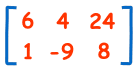（这矩阵有2行和3列）

## 加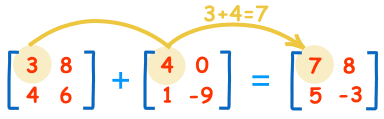3+4=7 8+0=8 4+1=5 6-9=-3

## 负矩阵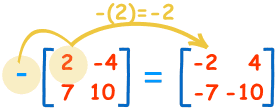-(2)=-2 -(-4)=+4 -(7)=-7 -(10)=-10

## 减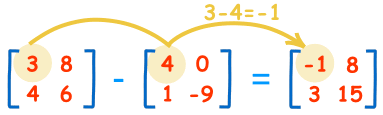3-4=-1 8-0=8 4-1=3 6-(-9)=15

## 乘以常数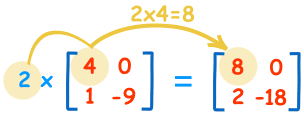2×4=8 2×0=0 2×1=2 2×-9=-18

## 除法

A/B = A × (1/B) = A × B-1

。。。。。。去逆矩阵了解更多。

## 矩阵转置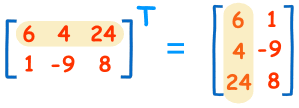## 记号法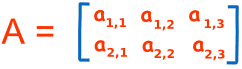• 行 从 左至右
• 列 从 上至下

### 例子：

 B =b1,1 = 6 （在行 1，列 1 的元素是 6）

b1,3 = 24 （在行 1，列 3 的元素是 24）

b2,3 = 8 （在行 2，列 3 的元素是 8）